# Height And Distance: Application In Trigonometry

The topic heights and distance is one of the applications of Trigonometry, which is extensively used in real-life. The words height and distance are frequently used in the trigonometry while dealing with its applications. In the height and distances application of trigonometry, the following concepts are included:

• Measuring the heights of towers or big mountains
• Determining the distance of the shore from the sea
• Finding the distance between two celestial bodies

It should be noted that finding the height of bodies and distances between two objects is one of the most important applications of trigonometry.

## What are Heights and Distances?

The most significant definitions that are used when dealing with heights and distances are given as:

• Definition 1: – Line of Sight –  The line which is drawn from the eyes of the observer to the point being viewed on the object is known as the line of sight.
• Definition 2:  – Angle of Elevation – The angle of elevation of the point on the object (above horizontal level) viewed by the observer is the angle which is formed by the line of sight with the horizontal level.
• Definition 3: – Angle of Depression – The angle of depression of the point on the object (below horizontal level) viewed by the observer is the angle which is formed by the line of sight with the horizontal level.

Also, check:

## How to Find Heights and Distances?

To measure heights and distances of different objects, we use trigonometric ratios. For example, in fig.1, a guy is looking at the top of the lamppost. AB is horizontal level. This level is the line parallel to ground passing through the observer’s eyes. AC is known as the line of sight. ∠A is called the angle of elevation. Similarly, in fig. 2, PQ is the line of sight, PR is the horizontal level and ∠P is called the angle of depression.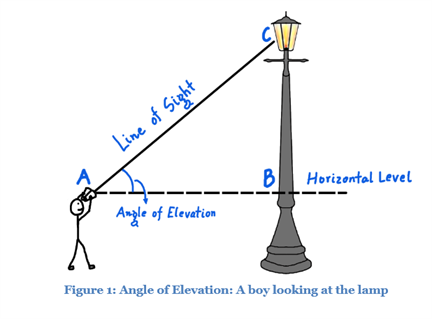Angle of Elevation in Height And Distance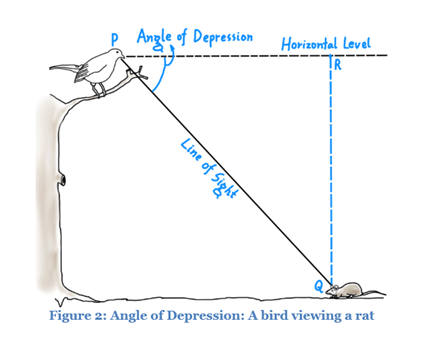Angle of Depression in Height And Distance

The angles of elevation and depression are usually measured by a device called Inclinometer or Clinometer.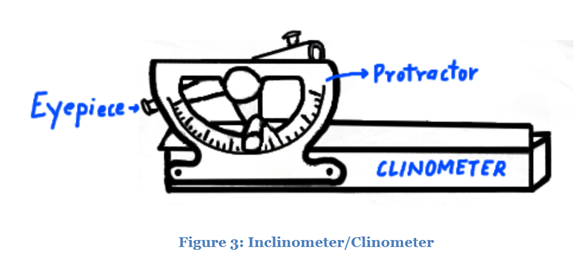Inclinometer for Measuring Angles of Elevation and Depression

To solve height and distance problems, trigonometric ratios for standard angles (Table 1) should be remembered.

Table 1: Trigonometric Ratios of Standard Angles

 ∠C 0° 30° 45° 60° 90° sin C 0 ½ 1/√2 √3/2 1 cos C 1 √3/2 1/√2 ½ 0 tan C 0 1/√3 1 √3 Not Defined cosec C Not Defined 2 √2 2/√3 1 sec C 1 2/√3 √2 2 Not Defined cot C Not Defined √3 1 1/√3 0

Solve a question based on the above topic for better understanding of this topic.

### Heights and Distances Formulas

In this section, you can see three different cases when solving height and distance questions.

Case 1: In this case, we can observe the following:

• Height of a tower, hill or building
• Distance of an object from the foot of the tower, hill or building and sometimes shadow of them
• Angle of elevation or the angle of depression

Any two of the above three parameters will be provided in the question. This type of problems can be solved using the formulas given below.

In right triangle ABC,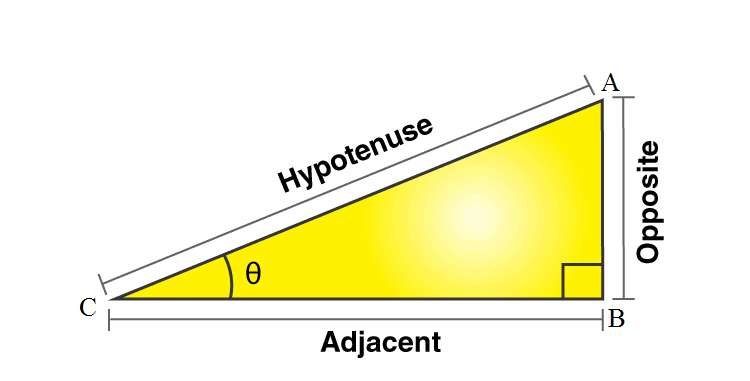sin θ = Opposite/Hypotenuse = AB/AC
cos θ = Adjacent/Hypotenuse = BC/AC
tan θ = Opposite/Adjacent = AB/BC

Case 2: In this case, we can deal with different illustrations. One of the commonly solved problems is about the movement of an observer. If the observer moves towards the objects like a tower, building, hill, etc., then the angle of elevation increases. The angle of elevation decreases when the observer moves away from the object. Here, the distance moved by the observer can be found using the formula given below:

In right triangle given below, d is the distance between C and D.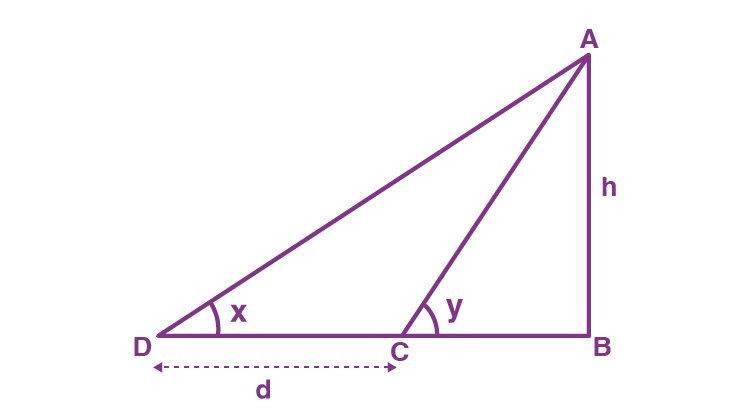d = h(cot x – cot y)

Case 3: There is another case where two different situations happening at the same. In this case, we get similar triangles with the same angle of elevation or angle of depression. These type of problems can be solved with the help of formulas related to similar triangles.

In right triangle ABC, DE || AB,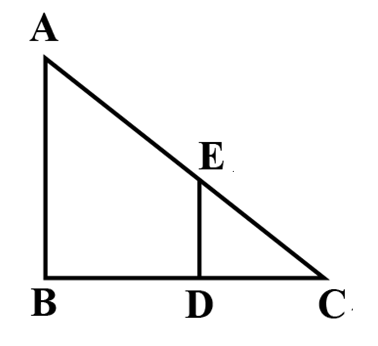Here, triangles ABC and EDC are similar.
Using Thales or BPT theorem we can write the ratio of sides as:
AB/ED = BC/DC

The above three formulas are used to solve majority of the heights and distances problems. Apart from these cases, there some other types of constructions could be drawn based on the given scenario. However, the combination of these formulas are used to solve any given problem related to the heights and distances in trigonometry.

#### For More Information On Trigonometry – Measuring Heights And Distances, Watch The Below Video: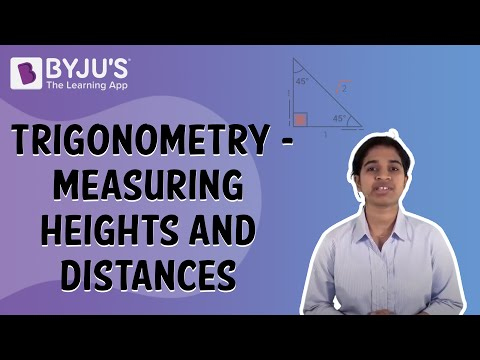### Heights and Distances Example Question

Question: An aeroplane is flying h meters above the ground. At a particular instant, the angle of elevation of the plane from the eyes of a boy sitting on the ground is 60°. After some time, the angle of elevation changed to 30°. Find the distance covered by the plane during that time assuming it travelled in a straight line.

Solution:

The scenario explained in the question can be drawn as shown in the figure.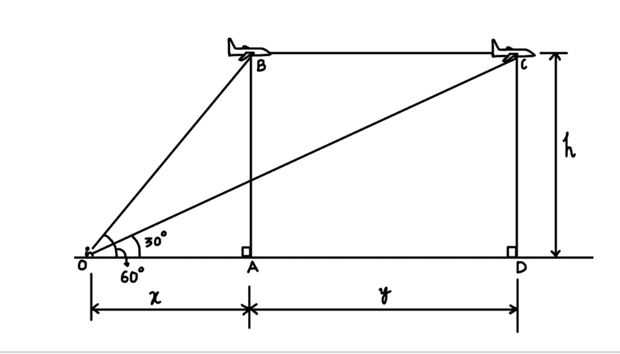Height And Distance Example Question

In ∆ OAB,

tan 60° = AB/OA

√3 = h/x

x = h/√3

In ∆ OCD,

tan 30° = CD/OD

1/√3 = h/(x+y)

x + y = √3h

Distance travelled by plane = AD = y

(x + y) − x = √3h − h/√3

y = (2/√3)h

So, if the aeroplane is flying h meters above the ground, it would travel for (2/√3) h meters as the angle of elevation changes from 60° to 30 °.

### Heights and Distances Practice Problems

1. From a point on a bridge across a river, the angles of depression of the banks on opposite sides of the river are 30° and 45°, respectively. If the bridge is at a height of 3 m from the banks, find the width of the river.
2. The angles of elevation of the top of a tower from two points at a distance of 4 m and 9 m from the base of the tower and in the same straight line with it are complementary. Prove that the height of the tower is 6 m.
3. From a balloon vertically above a straight road, the angles of depression of two cars at an instant are found to be 45° and 60°. If the cars are 100 m apart, find the height of the balloon.
4. The lower window of a house is at a height of 2 m above the ground and its upper window is 4 m vertically above the lower window. At certain instant the angles of elevation of a balloon from these windows are observed to be 60° and 30°, respectively. Find the height of the balloon above the ground.

### More Topics Related to Height and Distances:

Keep visiting BYJU’S for more such important maths articles. Also, download BYJU’S – The Learning App to learn maths in a more engaging and effective way.

Quiz on Height and Distance